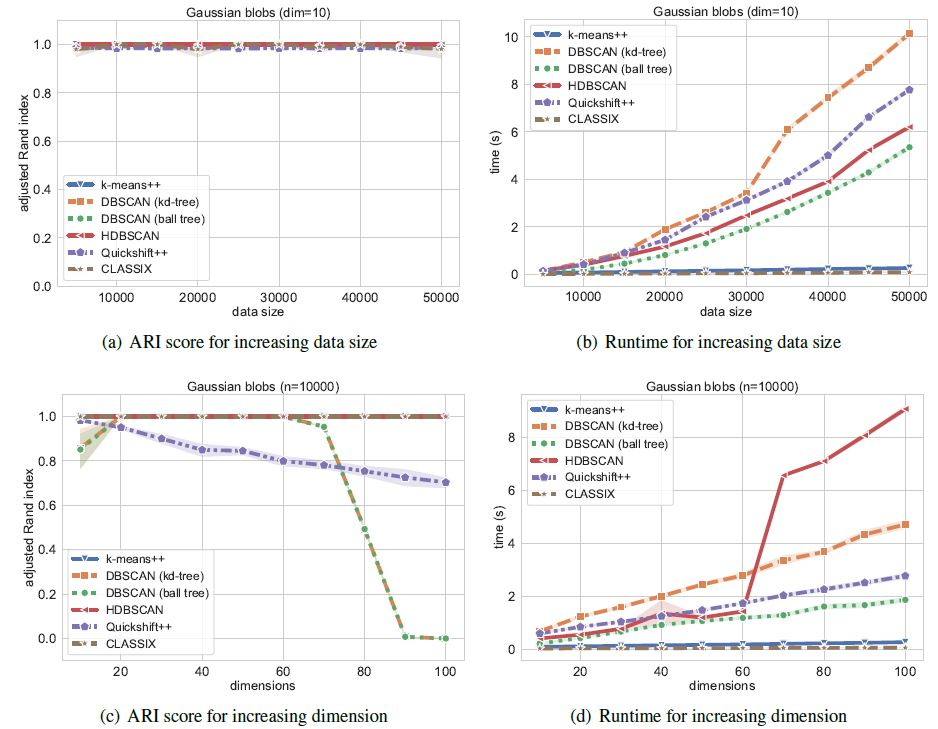# Performance Comparison

Here, we compare CLASSIX with the most typical and well-used density clustering algorithms DBSCAN, HDBSCAN and Quickshift++. We perform this experiment on Kamil dataset, and synthetic Gaussian blobs clusters.

## Built-in Data

```import time
import hdbscan
import warnings
import numpy as np
import pandas as pd
from sklearn import metrics
from sklearn.cluster import DBSCAN
import matplotlib.pyplot as plt
from classix import CLASSIX, load_data
```

Then we load the data:

```data = loadData('vdu_signals') # load the data
print(data.shape) # (2028780, 2)
```

This data has more than 2 million objects, which is very challenging for most clustering algorithm. Our experiments were runing on the environment settings:

• Dell PowerEdge R740 Server

• 2x Intel Xeon Silver 4114 2.2G (total 20 cores, 40 threads)

• 2x NVIDIA Tesla P100 16GB GPU

• 1.5 TB RAM (=1536 GB RAM)

• 1.8 TB disk space (expandable)

The DBSCAN, HDBSCAN, Quickshift++ fail in this experiment (runtime > 24 hr) while CLASSIX use around 1.2 seconds for clustering of whole data. Therefore, to compare the the four algorithms (ensure they can finish clustering within a day), we need to preprocess the data for downsampling:

```# This block of code is provided by Kamil Oster
final_len = 100000
outliers_position = np.where(X_pca[:,0] > 7.5)
no_outliers_position = np.delete(np.arange(0, len(X_pca[:,0])), outliers_position, axis=0)

outlier_len = len(outliers_position)
data_no_outliers_length = int(final_len - outlier_len)

data_outliers = X_pca[outliers_position, :]
data_no_outliers = np.delete(X_pca, outliers_position, axis=0)

random_integers = np.arange(0, len(no_outliers_position))
np.random.shuffle(random_integers)

data_no_outliers_out = data_no_outliers[random_integers[data_no_outliers_length:],:]
data_no_outliers =  data_no_outliers[random_integers[:data_no_outliers_length],:]

X = np.concatenate((data_no_outliers, data_outliers))
print(X.shape) # (100000, 2)
```

Cause other clustering algorithms almost cannot complete this clustering on the full data. So we employ CLASSIX clustering on the whole data while employing other clustering algorithms on down-sampling data, and get their average runtime for comparison:

```sample_size = 10 # each algorithm's running repeats for 10 times

sum_time = 0
timing = []

for i in range(sample_size):
st = time.time()
dbscan = DBSCAN(eps=0.7, min_samples=6)
dbscan.fit(X)
et = time.time()
sum_time = sum_time + et - st

timing.append(sum_time/sample_size)
print("Average consume time: ", sum_time/sample_size)
plt.figure(figsize=(24,10))
plt.scatter(X[:,0], X[:,1], c=dbscan.labels_, cmap='jet')
plt.tick_params(axis='both',  labelsize=15)
plt.title('DBSCAN',  fontsize=20)
plt.savefig('DBSCAN.png', bbox_inches='tight')
plt.show()

sum_time = 0
for i in range(sample_size):
st = time.time()
_hdbscan = hdbscan.HDBSCAN(min_cluster_size=1000, core_dist_n_jobs=1)
hdbscan_labels = _hdbscan.fit_predict(X)
et = time.time()
sum_time = sum_time + et - st

timing.append(sum_time/sample_size)
print("Average consume time: ", sum_time/sample_size)
plt.figure(figsize=(24,10))
plt.scatter(X[:,0], X[:,1], c=hdbscan_labels, cmap='jet')
plt.tick_params(axis='both',  labelsize=15)
plt.title('HDBSCAN',  fontsize=20)
plt.savefig('HDBSCAN.png', bbox_inches='tight')
plt.show()

sum_time = 0
for i in range(sample_size):
st = time.time()
quicks = QuickshiftPP(k=800, beta=0.7)
quicks.fit(X.copy(order='C'))
quicks_labels = quicks.memberships
et = time.time()
sum_time = sum_time + et - st

timing.append(sum_time/sample_size)
print("Average consume time: ", sum_time/sample_size)
plt.figure(figsize=(24,10))
plt.scatter(X[:,0], X[:,1], c=quicks_labels, cmap='jet')
plt.tick_params(axis='both',  labelsize=15)
plt.title('Quickshift++',  fontsize=20)
plt.savefig('Quickshiftpp.png', bbox_inches='tight')
plt.show()

sum_time = 0
for i in range(sample_size):
st = time.time()
clx = CLASSIX(sorting='pca', radius=0.3, verbose=0,  group_merging='distance')
clx.fit_transform(data)
et = time.time()
sum_time = sum_time + et - st

timing.append(sum_time/sample_size)
print("Average consume time: ", sum_time/sample_size)
plt.figure(figsize=(24,10))
plt.scatter(data[:,0], data[:,1], c=clx.labels_, cmap='jet')
plt.tick_params(axis='both',  labelsize=15)
plt.title('CLASSIX',  fontsize=20)
plt.savefig('CLASSIX.png', bbox_inches='tight')
plt.show()
```We can simply visualize the runtime:

```import seaborn as sns

data = pd.DataFrame()
data['clustering'] = ['DBSCAN', 'HDBSCAN', 'Quickshift++', 'CLASSIX']
data['runtime'] = timing

plt.figure(figsize=(12,6))
sns.set(font_scale=2, style="whitegrid")
ax = sns.barplot(x="clustering", y="runtime", data=data)
plt.savefig('results/runtime.png', bbox_inches='tight')
```

The runtime bar plot is as below, we can see that CLASSIX achieves the fastest speed even if it runs with the whole data.## Gaussian blobs

To provide another insight for clustering comparison with respect to runtime, we compare these algorithms by fixing optimal parameter setting on synthetic Gaussian blobs data with increasing size and dimension. So as to obtain a fair comparison of their runtime, we hope the clustering accuracy for all algorithms remains the same as much as possible as the data change. On the other hand, this experiment manifests the sensitivity of parameter settings to environmental settings. This Gaussian blobs test can be referred to in CLASSIX’s paper. The test is referenced from https://hdbscan.readthedocs.io/en/latest/performance_and_scalability.html.As we see from the figure, CLASSIX compares favorably against other algorithms while achieving the fastest speed and stable runtime among them.